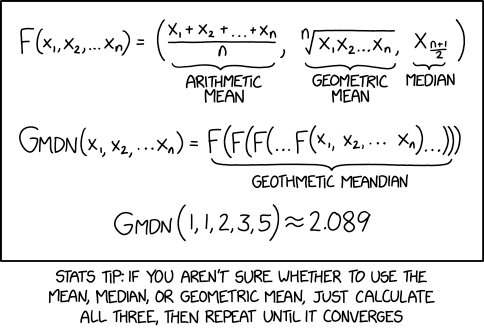## meanderingA bit of a misunderstanding from Randall Munroe and then some: the function F returns a triplet, hence G should return a triplet as well. Even if the limit does return three identical values. And he should have also included the (infamous) harmonic mean! And the subtext (behind the picture) mentions random forest statistics, using every mean one can think of and dropping those that are doing worse, while here all solutions return the same value, hence do not directly discriminate between the averages (and there is no objective function to create the nodes in the trees, &tc.).

Here is a test R code including the harmonic mean:

```xkcd=function(x)c(mean(x),exp(mean(log(x))),median(x),1/mean(1/x))
xxxkcd=function(x,N=10)ifelse(rep(N==1,4),xkcd(x),xxxkcd(xkcd(x),N-1))
xxxkcd(rexp(11))
 1.018197 1.018197 1.018197 1.018197
```

### One Response to “meandering”

1.meandering | R-bloggers Says:

[…] article was first published on R – Xi'an's Og, and kindly contributed to R-bloggers]. (You can report issue about the content on this page here) […]

This site uses Akismet to reduce spam. Learn how your comment data is processed.